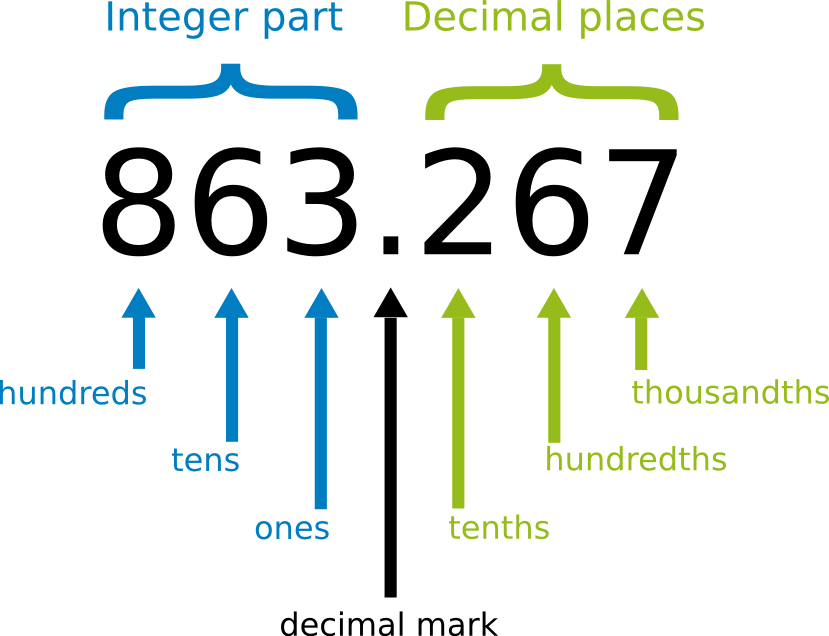Decimal fractions are numbers written in decimal notation. They are a very common way of displaying fractions in a compact manner. You will encounter them everywhere in your daily life: on price tags, sizes or on the display of your calculator.

Examples:

• $15.731$ is a decimal fraction. If written as a fraction it would look like this: $\dfrac {15731}{1000}$

• $7.50\,$ are $7\,$ and $50\, \mathrm {ct}$; definitely no one would write it as $\dfrac {15}{2} \,$.

• A quarter inch written as a decimal fraction is $0.25 \, \mathrm {in}$.

## Structure of decimal fractions

Every decimal fraction is constructed in the following way:
integer - decimal mark - decimal places:

• The decimal mark - written like a full stop - separates the integer part from the decimal places.
• In front of the decimal mark the integer part is written.
(Ones, tens, hundreds etc.)
• After the decimal mark the decimal places are written.
(tenths, hundredths, thousandths etc.)The decimal fraction shown in the figure will look like this when written in detail: $$863.267= 863+ \dfrac {2}{10} +\dfrac{6}{100} + \dfrac{7}{1000}$$

### Decimal fractions in the place value table

Decimal fractions like $863.267$ can be easily entered into the place value table if you expand it to include tenths, hundredths, thousandths etc.:

Th

H

T

O

.

t

h

th

0

8

6

3

.

2

6

7

## Number of decimal places in a decimal fraction

Essentially, a decimal fraction can possess any number of decimal places.
You need to distinguish between three different cases:

### 1. Terminating decimal fractions

In this case there is a finite number of decimal places after the decimal mark.

Examples:

• $190.4$
• $0.045000$
(Comment: Zeros at the end of a decimal fraction do not change its value; $0.045000$ is the same as $0.045$)
• $235.678554467$

### 2. Periodic decimal fractions

These decimal fractions possess an infinite number of decimal places after the decimal mark, but after a certain point there is an infinitely-repeated digit sequence.

This digit sequence is called the repetend of the decimal fraction and indicated by drawing a horizontal line above it.

Examples:

• $0.\overline {3}=0.3333333333…$
• $0.1\overline {6}=0.16666666….$
• $0.\overline {16}=0.1616161616….$
• $245.0674\overline {45698}=245.0674456984569845698…$

(Comment: A decimal fraction with a repetend "in between" (for example: $8. \overline {0} 21$) does not make any sense, since you would have to wait for the end of an infinite number of zeros before putting the $21$.)

### 3. Non-terminating non-periodic decimal fractions

If you tried to write an irrational number like $\sqrt {2}$ or $\pi$ as a decimal, you would end up with a number that has an infinite number of decimal places but no repeating sequence.

Examples:

• $\sqrt {2} =1.41421 35623 73095 04880 16887 24209 69807 85696 71875 37694… \approx1.41$
• $\pi = 3.1415926…\approx3.14$